## Social Question# Can you find a second relationship between 3 consecutive Fibonacci numbers?

Asked by LostInParadise (29660) December 19th, 2011

The Fibonacci series has a long history in mathematics. It has a curious habit of turning up in various places in nature.

The series is defined very simply. The first two members are 1 and 1. Each subsequent member is defined as the sum of the previous two, so the first few terms are:
1 1 2 3 5 8 13 21 34.

Given 3 consecutive Fibonacci numbers, f(n), f(n+1) and f(n+2), the defining relationship between them is f(n+2) = f(n) + f(n+1). See if you can discover another relationship. The equation varies slightly, depending on whether n is even or odd.

Observing members: 0Composing members: 0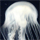I literally cannot visualize any of this.

whitetigress (3129)“Great Answer” (0) Flag as…Do you mean that you do not see how the series is generated?
1+1 =2, giving 1 1 2
1+2 = 3 giving 1 1 2 3
2+3=5 1 1 2 3 5
and so on.

LostInParadise (29660)“Great Answer” (0) Flag as…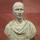1,2,3 are ordinal numbers.

FireMadeFlesh (16583)“Great Answer” (0) Flag as…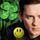I won’t give it away. But here’s a hint. .Take two neighboring numbers in the series and divide the first by the second, for example: ⅔= .666 3/5= .60 5/8= .625 8/13= .615 As you work your way to infinity you will see they converge on a truly golden number. You can work magic with two golden ratios next to each other.

LuckyGuy (40650)“Great Answer” (2) Flag as…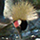Ratio between them converges to phi.

6rant6 (13697)“Great Answer” (0) Flag as…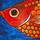f(n+2)*f(n) – f(n+1)*f(n+1) = (-1)exp(n)
That looks a bit clumsy, but if you multiply the largest by the smallest and take away the square of the middle number you will always get either +1 or -1.

flutherother (32727)“Great Answer” (3) Flag as…That’s it!
For extra credit, you can do an inductive proof.

The formula can be derived from a more general result, which I learned about from the section on Fibonacci in Strange Curves, which is a really good popular math book.

LostInParadise (29660)“Great Answer” (3) Flag as…Assume it is true for k then Fk+2Fk-F2k+1 = (-1)k
And Fk+2(Fk+2-Fk+1)-F2k+1= (-1)k
F2k+2 – (Fk+2.Fk+1)-F2k+1 = (-1)k
F2k+2—(Fk+2+Fk+1)Fk+1=(-1)k
F2k+2 –Fk+3Fk+1 =(-1)k
Reversing the signs Fk+3Fk+1-F2k+2 =(-1)k+1
Therefore it is true for k+1

flutherother (32727)“Great Answer” (1) Flag as…Here is the way I did it.
Given that f(k+2)f(k) – f^2(k+1) = (-1)^k
We want to show that f(k+3)f(k+1) -f^2(k+2) = (-1)^(k+1)

f(k+3)f(k+1) -f^2(k+2) =
(f(k+2) + f(k+1))f(k+1) – f^2(k+1) =
f^2(k+1) + f(k+2)f(k+1) – f^2(k+1) = (using the inductive assumption)
f(k+2)f(k) – (-1)^k + f(k+2)f(k+1) – f^2(k+2) =
f(k+2)f(k) + (-1)^(k+1) + f(k+2)(f(k+1) – f(k+2)) =
f(k+2)f(k) + (-1)^(k+1) + f(k+2)(-f(k)) =
-1(k+1)

LostInParadise (29660)“Great Answer” (1) Flag as…